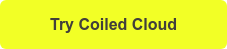August 20, 2021

This article explains how to redistribute data among partitions in a Dask DataFrame with repartitioning. Analyses run slower when data is unevenly distributed across partitions, and repartitioning can smooth out the data and provide a significant performance boost.

Dask DataFrames consist of partitions, each of which is a pandas DataFrame. Dask performance will suffer if there are lots of partitions that are too small or some partitions that are too big. Repartitioning a Dask DataFrame solves the issue of “partition imbalance”.

Let’s start with some simple minimal complete verifiable examples (MCVE) repartition examples to get you familiar with the repartition syntax.

We’ll then illustrate the performance gains from repartitioning a 63 million row dataset. This example demonstrates how the small file problem causes a computation to run 18 times slower and how this can be fixed with repartitioning.

## Simple examples

Let’s create a Dask DataFrame with six rows of data organized in three partitions.

```import pandas as pd

df = pd.DataFrame({"nums":[1, 2, 3, 4, 5, 6], "letters":["a", "b", "c", "d", "e", "f"]})
ddf = dd.from_pandas(df, npartitions=3)
```

Print the content of each DataFrame partition.

```for i in range(ddf.npartitions):
print(ddf.partitions[i].compute())

nums letters
0     1       a
1     2       b
nums letters
2     3       c
3     4       d
nums letters
4     5       e
5     6       f
```

Repartition the DataFrame into two partitions.

```ddf2 = ddf.repartition(2)

for i in range(ddf2.npartitions):
print(ddf2.partitions[i].compute())

nums letters
0     1       a
1     2       b
nums letters
2     3       c
3     4       d
4     5       e
5     6       f
```

`repartition(2)` causes Dask to combine partition 1 and partition 2 into a single partition. Dask’s repartition algorithm is smart to coalesce existing partitions and avoid full data shuffles.

You can also increase the number of partitions with repartition. Repartition the DataFrame into 5 partitions.

```ddf5 = ddf.repartition(5)

for i in range(ddf5.npartitions):
print(ddf5.partitions[i].compute())

nums letters
0     1       a
nums letters
1     2       b
nums letters
2     3       c
nums letters
3     4       d
nums letters
4     5       e
5     6       f```

In practice, it’s easier to repartition by specifying a target size for each partition (e.g. 100 MB per partition). You want Dask to do the hard work of figuring out the optimal number of partitions for your dataset. Here’s the syntax for repartitioning into 100MB partitions.

```ddf.repartition(partition_size="100MB")
```

## Example of small file problem

Let’s illustrate the small file problem and then explain how repartitioning can eliminate an excessive number of small files.

Suppose you have two years of data, one row per second, with the following structure:

That’s 63 million rows of data.

When the data is stored in 104 CSV files (41 MB each), it takes 16.6 seconds to get the number of unique values in the name column.

When the data is stored in 17,496 tiny CSV files (248 KB each), it takes a whopping 4 minutes and 16 seconds to perform the same computation. Having too many files causes the computation to run 15 times slower!

When the tiny CSV files are repartitioned into 100 partitions and written out as Parquet files, then the count operation can be performed in 4 seconds. Tiny files should be eliminated via repartitioning, so Dask can perform computations more efficiently.

See this notebook for the full benchmarking analysis with computations to support the results. Here’s the conda environment you can use to run the notebook, as described in the project README.

## When to repartition

Of course, repartitioning isn’t free and takes time. The cost of performing a full data shuffle can outweigh the benefits of subsequent query performance.

You shouldn’t always repartition whenever a dataset is imbalanced. Repartitioning should be approached on a case-by-case basis and only performed when the benefits outweigh the costs.

## Common causes of partition imbalance

Filtering is a common cause of DataFrame partition imbalance.

Suppose you have a DataFrame with a first_name column and the following data:

• Partition 0: Everyone has a first_name “Allie”
• Partition 1: Everyone has first_name “Matt”
• Partition 2: Everyone has first_name “Sam”

If you filter for all the rows with first_name equal to “Allie”, then Partition 1 and Partition 2 will be empty. Empty partitions cause inefficient Dask execution. It’s often wise to repartition after filtering.

## Next steps

This post has shown you how to fix DataFrame partition imbalance with repartitioning.

Repartitioning can be costly because it requires data to be shuffled. The overall performance gains from repartitioning should be measured on a case-by-case basis. The performance gains from having data evenly distributed across DataFrame partitions may be outweighed by the cost of performing the shuffle.

You’re well equipped to determine when it’s best to run this important performance optimization after learning about repartitioning in this post.

Thanks for reading. If you’re interested in trying out Coiled Cloud, which provides hosted Dask clusters, docker-less managed software, and one-click deployments, you can do so for free today when you click below.